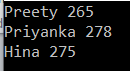# Linq Any in C#

## Linq Any in C# with Examples

In this article, I am going to discuss the Linq Any in C# with examples. Please read our previous article before proceeding to this article where we discussed the LINQ ALL Operator with some different kinds of examples. As part of this article, we are going to discuss the following pointers.

1. What is Linq Any in C#?
2. Multiple examples using both Method and Query syntax.
##### What is Linq Any in C#?

The C# Linq Any Operator is used to check whether at least one of the elements of a data source satisfies a given condition or not. If any of the elements satisfy the given condition, then it returns true else return false.

It is also used to check whether a collection contains some data or not. That means it checks the length of the collection also. If it contains any data then it returns true else return false. There are two overloaded versions of this method is available. They are as follows.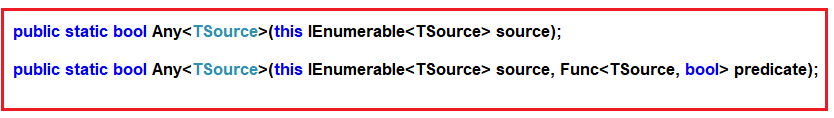As you can see from the above image, the first overloaded version of ANY extension method does not take any parameter while the other overloaded version takes a predicate as a parameter.

##### Example1: Using First Overloaded Version of Linq Any Operator

The following example returns true as the collection contains at least one element.

```using System;
using System.Linq;
namespace LINQDemo
{
class Program
{
static void Main(string[] args)
{
int[] IntArray = { 11, 22, 33, 44, 55 };

//Using Method Syntax
var ResultMS = IntArray.Any();

//Using Query Syntax
var ResultQS = (from num in IntArray
select num).Any();

Console.WriteLine("Is there any element in the collection : " + ResultMS);
}
}
}```

Output: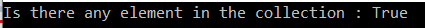##### Example2: Using second Overloaded version of C# Linq Any Operator

The following program returns false as there is no element that is less than 10.

```using System;
using System.Linq;
namespace LINQDemo
{
class Program
{
static void Main(string[] args)
{
int[] IntArray = { 11, 22, 33, 44, 55 };
var Result = IntArray.All(x => x > 10);
Console.WriteLine("Is All Numbers are greater than 10 : " + Result);
}
}
}
```

Output: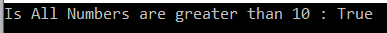##### Example3:

The following example returns true as some of the names are greater than 5 characters.

```using System;
using System.Linq;
namespace LINQDemo
{
class Program
{
static void Main(string[] args)
{
string[] stringArray = { "James", "Sachin", "Sourav", "Pam", "Sara" };

//Method Syntax
var ResultMS = stringArray.Any(name => name.Length > 5);

//Query Syntax
var ResultQS = (from name in stringArray
select name).Any(name => name.Length > 5);

Console.WriteLine("Is Any name with length  greater than 5 Characters : " + ResultMS);
}
}
}
```

Output: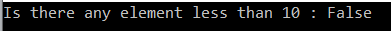##### Working with Complex Type:

We are going to work with the following Student and Subject class. So, create a class file with the name Student.cs and then copy and paste the following code.

```using System.Collections.Generic;
namespace LINQDemo
{
public class Student
{
public int ID { get; set; }
public string Name { get; set; }
public int TotalMarks { get; set; }
public List<Subject> Subjects { get; set; }

public static List<Student> GetAllStudnets()
{
List<Student> listStudents = new List<Student>()
{
new Student{ID= 101,Name = "Preety", TotalMarks = 265,
Subjects = new List<Subject>()
{
new Subject(){SubjectName = "Math", Marks = 80},
new Subject(){SubjectName = "Science", Marks = 90},
new Subject(){SubjectName = "English", Marks = 95}
}},
new Student{ID= 102,Name = "Priyanka", TotalMarks = 278,
Subjects = new List<Subject>()
{
new Subject(){SubjectName = "Math", Marks = 90},
new Subject(){SubjectName = "Science", Marks = 95},
new Subject(){SubjectName = "English", Marks = 93}
}},
new Student{ID= 103,Name = "James", TotalMarks = 240,
Subjects = new List<Subject>()
{
new Subject(){SubjectName = "Math", Marks = 70},
new Subject(){SubjectName = "Science", Marks = 80},
new Subject(){SubjectName = "English", Marks = 90}
}},
new Student{ID= 104,Name = "Hina", TotalMarks = 275,
Subjects = new List<Subject>()
{
new Subject(){SubjectName = "Math", Marks = 90},
new Subject(){SubjectName = "Science", Marks = 90},
new Subject(){SubjectName = "English", Marks = 95}
}},
new Student{ID= 105,Name = "Anurag", TotalMarks = 255,
Subjects = new List<Subject>()
{
new Subject(){SubjectName = "Math", Marks = 80},
new Subject(){SubjectName = "Science", Marks = 90},
new Subject(){SubjectName = "English", Marks = 85}
}
},
};

return listStudents;
}
}

public class Subject
{
public string SubjectName { get; set; }
public int Marks { get; set; }
}
}```
##### Example4:

Check whether any students having total marks greater than 250. As you can see excepts James all the students having a total mark greater than 250. So here it will give you the output as true.

```using System;
using System.Linq;
namespace LINQDemo
{
class Program
{
static void Main(string[] args)
{
//Using Method Syntax
bool MSResult = Student.GetAllStudnets().Any(std => std.TotalMarks > 250);

//Using Query Syntax
bool QSResult = (from std in Student.GetAllStudnets()
select std).Any(std => std.TotalMarks > 250);

Console.WriteLine(MSResult);
}
}
}
```

Output: False

##### Example5:

Now we need to fetch all the student details whose mark on any subject is greater than 80.

```using System;
using System.Linq;
namespace LINQDemo
{
class Program
{
static void Main(string[] args)
{
//Using Method Syntax
var MSResult = Student.GetAllStudnets()
.Where(std => std.Subjects.Any(x => x.Marks > 90)).ToList();

//Using Query Syntax
var QSResult = (from std in Student.GetAllStudnets()
where std.Subjects.Any(x => x.Marks > 90)
select std).ToList();

foreach (var item in QSResult)
{
Console.WriteLine(item.Name + " " + item.TotalMarks);
}